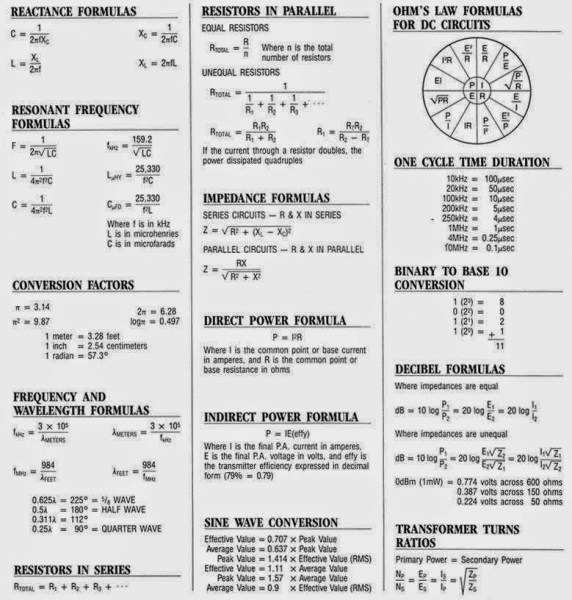Lets Make Tech

# Electrical Formulas

I saw this sheet floating around the internet. Very useful formula reference sheet.# Using The GMP Library

Here is some code for finding factorials using the GMP library.

-Really large numbers may take more time than you/your computer are willing/able to spend and that this is not multiple threads so don't go trying to break any records.

-Compile it with the tags it requires and you need to install libgmp3-dev

``````//When compiling include -lgmpxx and -lgmp and have sudo apt-get install libgmp3-dev
#include <iostream>
#include <gmpxx.h>
#include <fstream>
using namespace std;
int main()
{
ofstream fout;
fout.open("Yournumber.txt");
mpz_class Number, Temp1, ans, anstwo;
cout<<"Factorial Calculator\nEnter number: ";
cin>>Number;

if (Number==0){ans=0;}

else{ans=1;}

Temp1=Number;
for (int i=0;i<Number;i++)
{
ans=ans*Temp1;
Temp1=Temp1-1;
}
anstwo=ans;
for (int j=0;j<11;j++)
{
anstwo=ans*anstwo;
}

fout<<anstwo;
fout.close();
return 0;
}``````

Example of using the GMP library to find Fibonacci numbers.

``````//When compiling include -lgmpxx and -lgmp and have sudo apt-get install libgmp3-dev
#include <iostream>
#include <fstream>
#include <gmpxx.h>
using namespace std;
int main ()
{
ofstream fout;
fout.open("fibonacci_numbers.txt");
int amount, ans=0, temp1=1, temp2;         cout<<"How many?";        cin>>amount;
for (int i=0;i<amount;i++)
{
fout<<ans<<endl;
temp2=ans;
ans=ans+temp1;
temp1 = temp2;
}
}``````
Home